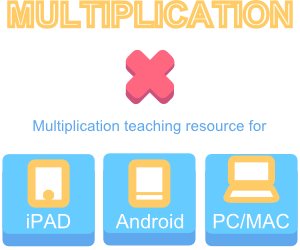## Resources for teaching and practising multiplication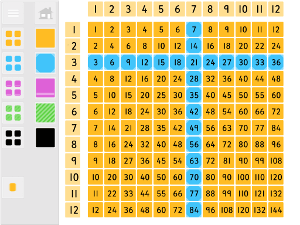#### Multiplication Grid

A multiplication grid for teaching times tables and as a reference for children is a useful classroom tool.  Hide, colour ...#### Carroll Diagram

This teaching resource is for both teaching how to use Carroll diagrams and for practising using them. There are two ...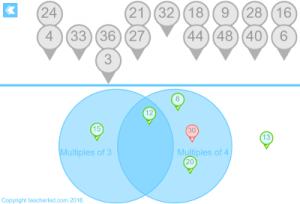#### Venn Chart

This resource has two sections. One is for children to practise sorting multiples on a Venn diagram.  This is timed ...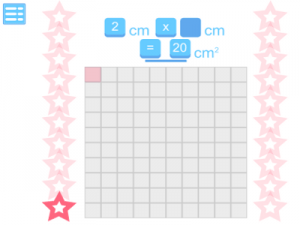#### Show The Area

Develop multiplication skills in the context of area of rectangles using this resource.  One number is missing from the question ...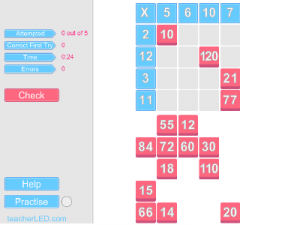#### Multiplication Grid

Teach and practise multiplication tables up to 12 x 12 on this teaching resource for IWB and mobile devices such ...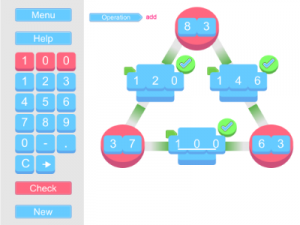#### Arithmagons

This teaching and learning resource uses the old favourite: Arithmagons.  The thought to produce an updated versions of this resource ...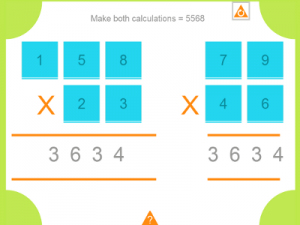#### Nine Digits

A mathematical based puzzle for IWB, iPad and Android that requires some careful numeracy thinking and some informed trial and ...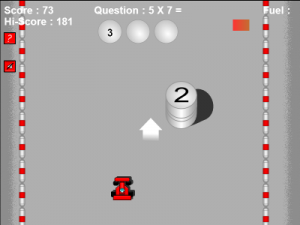#### Multiplication Sprint

This maths-practice game was originally for practising multiplication but now includes other arithmetic too The purpose of the game is ...